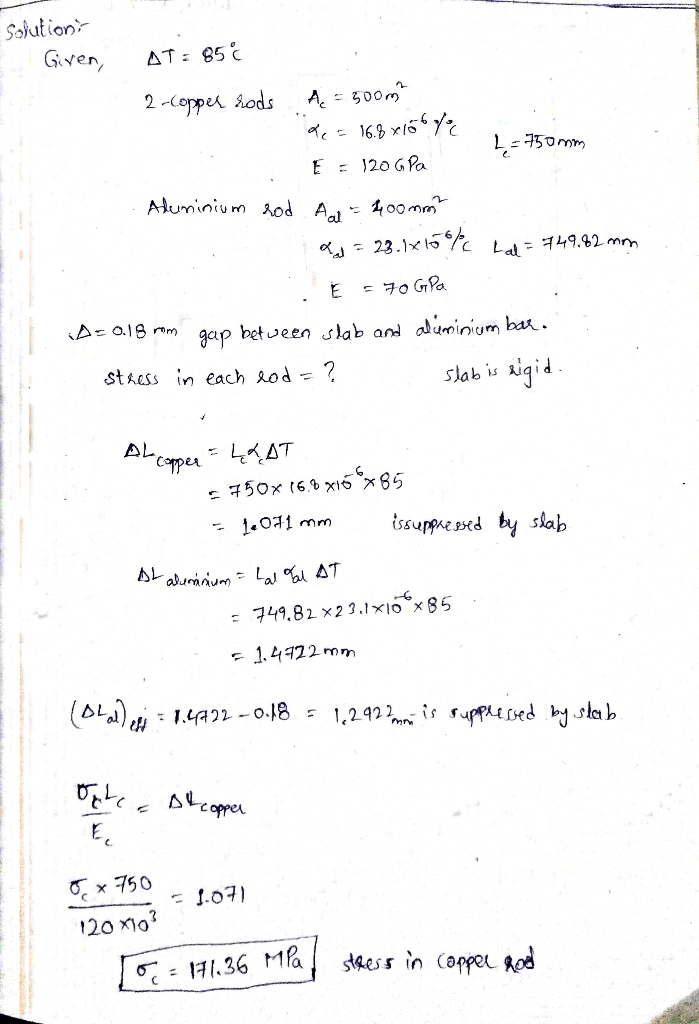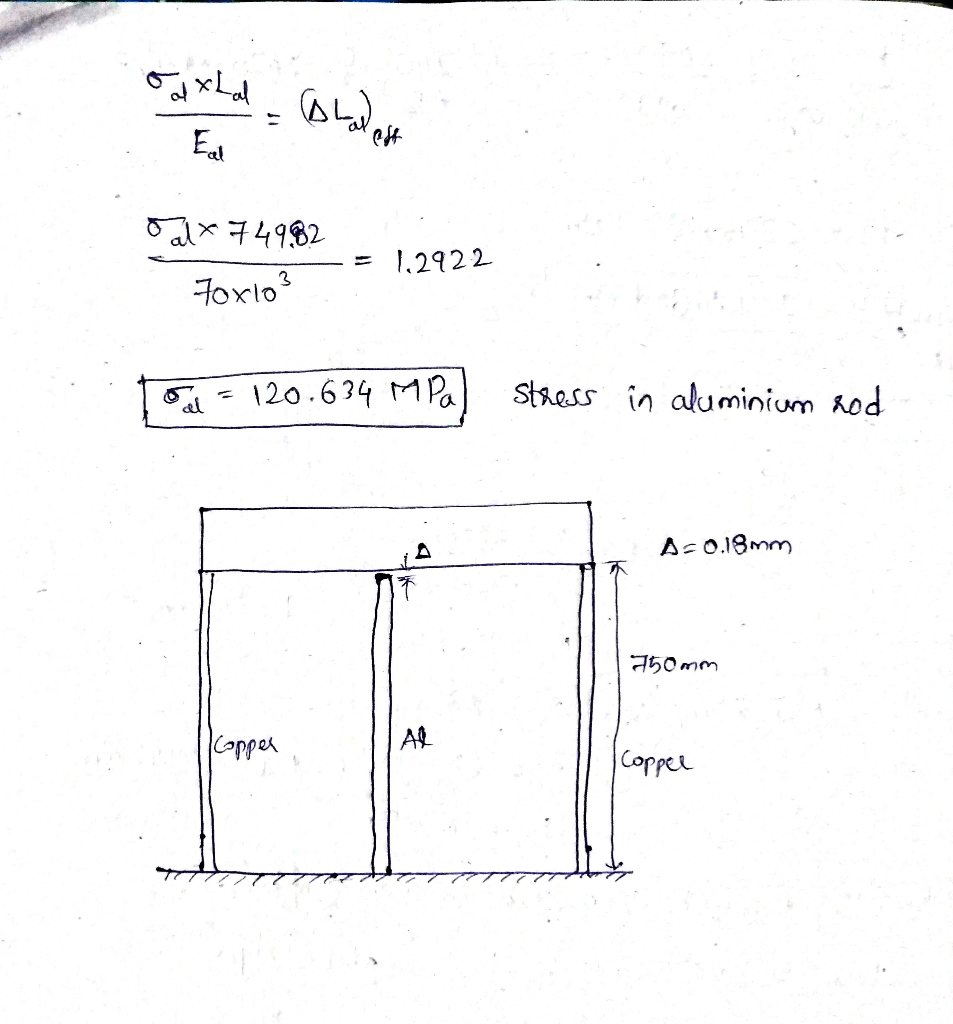# The rigid, horizontal slab is attached to two identical copper rods. There is a gap A = 0.18 mm between the middle bar, wh 7. The rigid, horizontal slab is attached to two identical copper rods. There is a gap A = 0.18 mm between the middle bar, which is made of aluminum, and the slab. Neglecting the mass of the slab, calculate the stress in each rod when the temperature in the assembly is increased by 85°C. Use the following data

Question-AnswerCategory: Strength of MaterialsThe rigid, horizontal slab is attached to two identical copper rods. There is a gap A = 0.18 mm between the middle bar, wh 7. The rigid, horizontal slab is attached to two identical copper rods. There is a gap A = 0.18 mm between the middle bar, which is made of aluminum, and the slab. Neglecting the mass of the slab, calculate the stress in each rod when the temperature in the assembly is increased by 85°C. Use the following data7. The rigid, horizontal slab is attached to two identical copper rods. There is a gap A = 0.18 mm between the middle bar, which is made of aluminum, and the slab. Neglecting the mass of the slab, calculate the stress in each rod when the temperature in the assembly is increased by 85°C. Use the following data